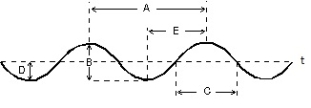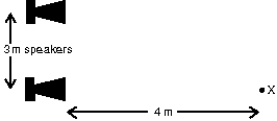Try out our new practice tests completely free!# Fundamentals of Physics Study Set 2

Physics & Astronomy

## Quiz 17 : Waves IIStudy FlashcardsLooking for Introductory Physics Homework Help?

## Quiz 17 :Waves IIDuring a time interval of exactly one period of vibration of a tuning fork, the emitted sound travels a distance:
Free
Multiple Choice

E

Tags
Choose question tagThis graph shows the position of an element of air as a function of time as a sound wave passes through it.Which letter corresponds to the period of the wave?Free
Multiple Choice

A

Tags
Choose question tagTwo small identical speakers are connected (in phase)to the same source.The speakers are 3 m apart and at ear level.An observer stands at X, 4 m in front of one speaker as shown.The sound she hears will be most intense if the wavelength is:Free
Multiple Choice

E

Tags
Choose question tagTwo waves are out of phase by half a wavelength.What is this in radians?
Multiple Choice
Tags
Choose question tagThe speed of a sound wave is determined by:
Multiple Choice
Tags
Choose question tagWhat is a wavefront?
Multiple Choice
Tags
Choose question tagThe longitudinal displacement of a mass element in a medium as a sound wave passes through it is given by s = sm cos (kx - ωt).Consider a sound wave of frequency 440 Hz and wavelength 0.75m.If sm = 12 µm, how long does it take an element of air to move from a displacement of 12 µm to a displacement of 0 µm?
Multiple Choice
Tags
Choose question tagA fire whistle emits a tone of 170 Hz.Take the speed of sound in air to be 340 m/s.The wavelength of this sound is about:
Multiple Choice
Tags
Choose question tagTwo sound waves are traveling through the same medium.They have the same amplitude, wavelength, and direction of travel.If the phase difference between them is 7π, the type of interference they exhibit is:
Multiple Choice
Tags
Choose question tagThe difference between transverse and longitudinal waves:
Multiple Choice
Tags
Choose question tagTake the speed of sound to be 340 m/s.A thunder clap is heard about 3 s after the lightning is seen.The source of both light and sound is:
Multiple Choice
Tags
Choose question tagThis graph shows the position of an element of air as a function of time as a sound wave passes through it.Which letter corresponds to the amplitude of the wave?Multiple Choice
Tags
Choose question tagThe longitudinal displacement of a mass element in a medium as a sound wave passes through it is given by s = sm cos (kx - ωt).Consider a sound wave of frequency 440 Hz and wavelength 0.75m.If sm = 12 µm, what is the displacement of an element of air located at x = 1.2 m at time t = 0.11 s?
Multiple Choice
Tags
Choose question tagTwo small identical speakers are connected (in phase)to the same source.The speakers are 3 m apart and at ear level.An observer stands at X, 4 m in front of one speaker as shown.If the amplitudes are not changed, the sound he hears will be least intense if the wavelength is:Multiple Choice
Tags
Choose question tagThe speed of sound in air is 340 m/s, and the density of air is 1.2 kg/m3.If the displacement amplitude of a 440-Hz sound wave is 10 µm, what is its pressure-variation amplitude?
Multiple Choice
Tags
Choose question tagWhich of the following properties of a sound wave determine its "pitch"?
Multiple Choice
Tags
Choose question tagAt points in a sound wave where the gas is maximally compressed, the pressure
Multiple Choice
Tags
Choose question tagThe bulk modulus of water is 2.2 x 109 Pa, and its density is 1.0 x 103 kg/m3.What is the speed of sound in water?
Multiple Choice
Tags
Choose question tagTwo waves are out of phase by half a wavelength.What is this in degrees?
Multiple Choice